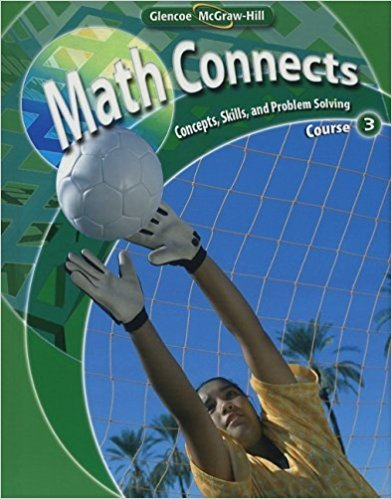×
×

# Solutions for Chapter 4: Math Connects: Concepts, Skills, and Problem Solving Course 3 0th Edition## Full solutions for Math Connects: Concepts, Skills, and Problem Solving Course 3 | 0th Edition

ISBN: 9780078740503Solutions for Chapter 4

Solutions for Chapter 4
4 5 0 357 Reviews
27
4
##### ISBN: 9780078740503

Since 9 problems in chapter 4 have been answered, more than 1155 students have viewed full step-by-step solutions from this chapter. Math Connects: Concepts, Skills, and Problem Solving Course 3 was written by and is associated to the ISBN: 9780078740503. Chapter 4 includes 9 full step-by-step solutions. This textbook survival guide was created for the textbook: Math Connects: Concepts, Skills, and Problem Solving Course 3, edition: 0. This expansive textbook survival guide covers the following chapters and their solutions.

Key Math Terms and definitions covered in this textbook
• Cholesky factorization

A = CTC = (L.J]))(L.J]))T for positive definite A.

• Cramer's Rule for Ax = b.

B j has b replacing column j of A; x j = det B j I det A

• Determinant IAI = det(A).

Defined by det I = 1, sign reversal for row exchange, and linearity in each row. Then IAI = 0 when A is singular. Also IABI = IAIIBI and

• Distributive Law

A(B + C) = AB + AC. Add then multiply, or mUltiply then add.

• Echelon matrix U.

The first nonzero entry (the pivot) in each row comes in a later column than the pivot in the previous row. All zero rows come last.

• Eigenvalue A and eigenvector x.

Ax = AX with x#-O so det(A - AI) = o.

• Fast Fourier Transform (FFT).

A factorization of the Fourier matrix Fn into e = log2 n matrices Si times a permutation. Each Si needs only nl2 multiplications, so Fnx and Fn-1c can be computed with ne/2 multiplications. Revolutionary.

• Incidence matrix of a directed graph.

The m by n edge-node incidence matrix has a row for each edge (node i to node j), with entries -1 and 1 in columns i and j .

• Indefinite matrix.

A symmetric matrix with eigenvalues of both signs (+ and - ).

• Length II x II.

Square root of x T x (Pythagoras in n dimensions).

• Linear transformation T.

Each vector V in the input space transforms to T (v) in the output space, and linearity requires T(cv + dw) = c T(v) + d T(w). Examples: Matrix multiplication A v, differentiation and integration in function space.

• Normal matrix.

If N NT = NT N, then N has orthonormal (complex) eigenvectors.

• Nullspace N (A)

= All solutions to Ax = O. Dimension n - r = (# columns) - rank.

• Outer product uv T

= column times row = rank one matrix.

• Partial pivoting.

In each column, choose the largest available pivot to control roundoff; all multipliers have leij I < 1. See condition number.

• Rank one matrix A = uvT f=. O.

Column and row spaces = lines cu and cv.

• Schur complement S, D - C A -} B.

Appears in block elimination on [~ g ].

• Schwarz inequality

Iv·wl < IIvll IIwll.Then IvTAwl2 < (vT Av)(wT Aw) for pos def A.

• Semidefinite matrix A.

(Positive) semidefinite: all x T Ax > 0, all A > 0; A = any RT R.

• Vandermonde matrix V.

V c = b gives coefficients of p(x) = Co + ... + Cn_IXn- 1 with P(Xi) = bi. Vij = (Xi)j-I and det V = product of (Xk - Xi) for k > i.

×